## Definition

Nesterov's gradient acceleration refers to a general approach that can be used to modify a gradient descent-type method to improve its initial convergence.

### The two-step iteration description

In this description, there are two intertwined sequences of iterates that constitute our guesses: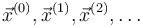$\vec{x}^{(0)}, \vec{x}^{(1)}, \vec{x}^{(2)}, \dots$$\vec{y}^{(0)}, \vec{y}^{(1)}, \vec{y}^{(2)}, \dots$

Explicitly, the sequences are intertwined as follows: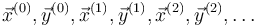$\vec{x}^{(0)}, \vec{y}^{(0)}, \vec{x}^{(1)}, \vec{y}^{(1)}, \vec{x}^{(2)}, \vec{y}^{(2)}, \dots$

We use parenthesized superscripts to denote the iteration stage, because the subscripts are reserved for use by the coordinates.

For the initialization, we set:$\vec{x}^{(0)} = \vec{y}^{(0)} = \mbox{Initial guess}$

The two-step iteration is as follows:

•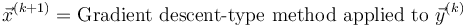$\vec{x}^{(k+1)} = \mbox{Gradient descent-type method applied to } \vec{y}^{(k)}$
•$\vec{y}^{(k+1)} = \vec{x}^{(k+1)} + m^{(k+1)}\left(\vec{x}^{(k + 1)} - \vec{x}^{(k)}\right)$

Note that the sign on the right side is not a typographical error:$\vec{y}^{(k+1)}$ is a non-convex combination of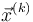$\vec{x}^{(k)}$ and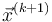$\vec{x}^{(k+1)}$. The value$m^{(k+1)}$ is a member of a predetermined sequence of real numbers in the interval$[0,1)$ that is independent of the specific problem. A typical expression that works is: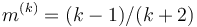$m^{(k)} = (k - 1)/(k + 2)$

This would yield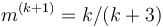$m^{(k+1)} = k/(k + 3)$.

### The description in terms of an approximating quadratic that is updated at each iteration

Fill this in later